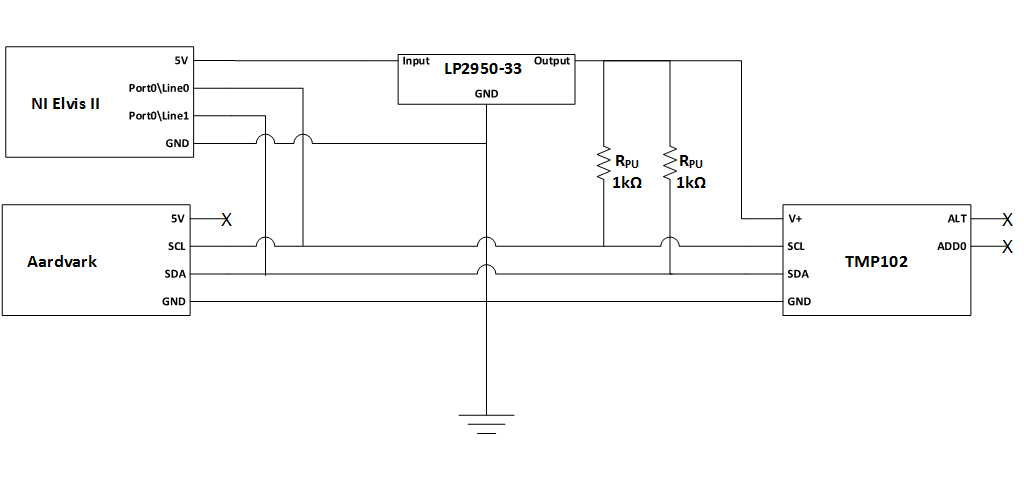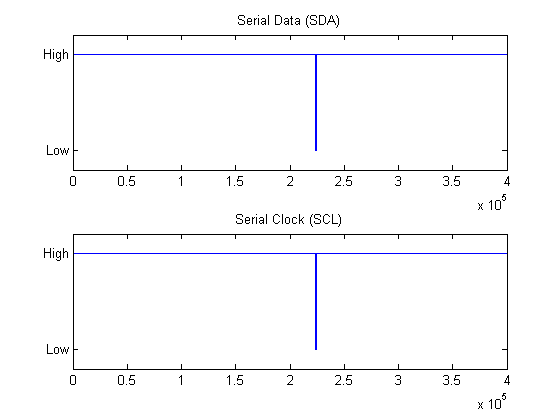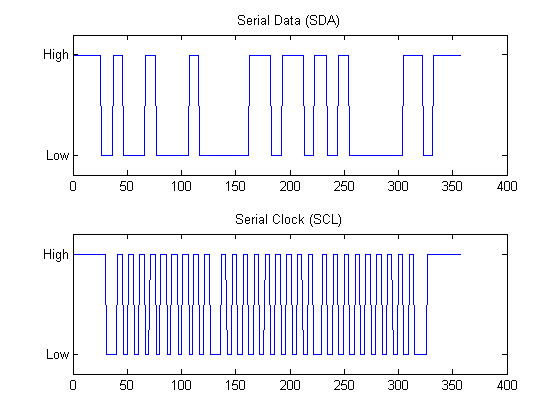Main Content

# Communicate with I2C Devices and Analyze Bus Signals Using Digital IO

Communicate with instruments and devices at the protocol layer as well as the physical layer. Use the I2C feature of Instrument Control Toolbox to communicate with a TMP102 temperature sensor, and simultaneously analyze the physical layer I2C bus communications using the clocked digital IO feature of Data Acquisition Toolbox.

Data Acquisition Toolbox and Instrument Control Toolbox are required.

### Hardware Configuration and Schematic

• Any supported National Instruments™ DAQ device with clocked DIO channels can be used (e.g., NI Elvis II)

• TotalPhase Aardvark I2C/SPI Host Adaptor

• TMP102 Digital Temperature Sensor with two-wire serial interface

The TMP102 requires a 3.3 V supply. Use a linear LDO (LP2950-33) to generate the 3.3 V supply from the DAQ device's 5 V supply line.

Alternative options include:

• Use an external power supply.

• Use an analog output channel from your DAQ device.### Connect to a TMP102 Sensor Using I2C Host Adaptor and Read Temperature Data

Wire up the sensor and verify communication to it using the I2C object from Instrument Control Toolbox.

```aa = instrhwinfo('i2c', 'aardvark'); % Get information about connected I2C hosts tmp102 = i2c('aardvark',0,hex2dec('48')); % Create an I2C object to connect to the TMP102 tmp102.PullupResistors = 'both'; % Use host adaptor pull-up resistors fopen(tmp102); % Open the connection data8 = fread(tmp102, 2, 'uint8'); % Read 2 byte data % One LSB equals 0.0625 deg. C temperature = ... (double(bitshift(int16(data8(1)), 4)) +... double(bitshift(int16(data8(2)), -4))) * 0.0625; % Refer to TMP102 data sheet to calculate temperature from received data fprintf('The temperature recorded by the TMP102 sensor is: %s deg. C\n',num2str(temperature)); fclose(tmp102); ```
```The temperature recorded by the TMP102 sensor is: 27.625 deg. C ```

### Acquire the Corresponding I2C Physical Layer Signals Using a DAQ Device

Use oversampled clocked digital channels from the NI Elvis (`Dev4`) to acquire and analyze the physical layer communications on the I2C bus.

Acquire SDA data on port 0, line 0 of your DAQ device. Acquire SCL data on port 0, line 1 of your DAQ device.

```dd = daq("ni"); addinput(dd,"Dev4","port0\line0","Digital"); % sda addinput(dd,"Dev4","port0\line1","Digital"); % scl ```

### Generate a Clock Signal for Use with the Digital Subsystem

Digital subsystems on NI DAQ devices do not have their own clock; they must share a clock with the analog subsystem or import a clock from an external subsystem. Generate a 50% duty cycle clock at 1 MHz using a `PulseGeneration` counter output, and set the input scan rate to match.

```pgChan = addoutput(dd,"Dev4","ctr1"),"PulseGeneration"); dd.Rate = 1e6; pgChan.Frequency = dd.Rate; ```

The clock is generated on the 'pgChan.Terminal' pin, allowing synchronization with other devices and viewing the clock on an oscilloscope. The counter output pulse signal is imported as a clock signal.

```disp(pgChan.Terminal); addclock(dd,"ScanClock","External",["Dev4/" pgChan.Terminal]); ```
```PFI13 ```

### Acquire the I2C Signals Using Clocked Digital Channels

Acquire data in the background from the SDA and SCL digital lines.

• Start the DataAcquisition in background mode

• Start the I2C operations

• Stop the DataAcquisition after I2C operations are complete

```start(dd, "continuous"); fopen(tmp102); data8 = fread(tmp102, 2, "uint8"); % One LSB equals 0.0625 deg. C temperature = (double(bitshift(int16(data8(1)), 4)) +... double(bitshift(int16(data8(2)), -4))) * 0.0625; fclose(tmp102); pause(0.1); stop(dd); myData = read(dd, "all"); ```
```Warning: Triggers and Clocks will not affect counter output channels. ```

Plot the raw data to see the acquired signals. Notice that lines are held high during idle periods. The next section shows how to find the start/stop condition bits and use them to isolate areas of interest in the I2C communication.

```figure("Name", "Raw Data"); subplot(2,1,1); plot(myData(:,1)); ylim([-0.2, 1.2]); ax = gca; ax.YTick = [0,1]; ax.YTickLabel = {'Low','High'}; title("Serial Data (SDA)"); subplot(2,1,2); plot(myData(:,2)); ylim([-0.2, 1.2]); ax = gca; ax.YTick = [0,1]; ax.YTickLabel = {'Low','High'}; title("Serial Clock (SCL)"); ```### Analyze the I2C Physical Layer Bus Communications

Extract I2C physical layer signals on the SDA and SCL lines.

```sda = myData(:,1)'; scl = myData(:,2)'; ```

Find all rising and falling clock edges.

```sclFlips = xor(scl(1:end-1), scl(2:end)); sclFlips = [1 sclFlips 1]; sclFlipIndexes = find(sclFlips==1); ```

Calculate the clock periods from the clock indices

```sclFlipPeriods = sclFlipIndexes(1:end)-[1 sclFlipIndexes(1:end-1)]; ```

Through inspection, observe that idle periods have SCL high for longer than 100 us. Since scan rate = 1MS/s, each sample represents 1 us. `idlePeriodIndices` indicate periods periods of activity within the I2C communication.

```idlePeriodIndices = find(sclFlipPeriods>100); ```

Zoom into the first period of activity on the I2C bus. For ease of viewing, include 30 samples of idle activity to the front and end of each plot.

```range1 = sclFlipIndexes(idlePeriodIndices(1)) - 30 : sclFlipIndexes(idlePeriodIndices(2) - 1) + 30; figure("Name", "I2C Communication Data"); subplot(2,1,1); plot(sda(range1)); ylim([-0.2, 1.2]); ax = gca; ax.YTick = [0,1]; ax.YTickLabel = {'Low','High'}; title("Serial Data (SDA)"); subplot(2,1,2); plot(scl(range1)); ylim([-0.2, 1.2]); ax = gca; ax.YTick = [0,1]; ax.YTickLabel = {'Low','High'}; title("Serial Clock (SCL)"); ```### Analyze Bus Performance Metrics

As a simple example analyze start and stop condition metrics, and I2C bit rate calculation.

• Start condition duration is defined as the time it takes for SCL to go low after SDA goes low.

• Stop condition duration is defined as the time it takes for SDA to go high after SCL goes high.

• Bit rate is calculated by taking the inverse of the time between 2 rising clock edges.

Start Condition: First SDA low, then SCL low

```sclLowIndex = sclFlipIndexes(idlePeriodIndices(1)); sdaLowIndex = find(sda(1:sclLowIndex)==1, 1, "last") + 1; % +1, flip is next value after last high startConditionDuration = (sclLowIndex - sdaLowIndex) * 1/s.Rate; fprintf('sda: %s\n', sprintf('%d ', sda(sdaLowIndex-1:sclLowIndex))); % Indexes point to next change, hence sclLowIndex includes flip to low fprintf('scl: %s\n', sprintf('%d ', scl(sdaLowIndex-1:sclLowIndex))); % subtract 1 from sdaLowIndex to see sda value prior to flip fprintf('Start condition duration: %d sec.\n\n', startConditionDuration); % count 5 pulses, 5 us. ```
```sda: 1 0 0 0 0 0 0 scl: 1 1 1 1 1 1 0 Start condition duration: 5.000000e-06 sec. ```

Stop Condition: First SCL high, then SDA high

```% flip prior to going into idle is the one we want sclHighIndex = sclFlipIndexes(idlePeriodIndices(2)-1); sdaHighIndex = find(sda(sclHighIndex:end)==1, 1, 'first') + sclHighIndex - 1; stopConditionDuration = (sdaHighIndex - sclHighIndex) * 1/s.Rate; fprintf('sda: %s\n', sprintf('%d ',sda(sclHighIndex-1:sdaHighIndex))); fprintf('scl: %s\n', sprintf('%d ',scl(sclHighIndex-1:sdaHighIndex))); fprintf('Stop condition duration: %d sec.\n\n', stopConditionDuration); ```
```sda: 0 0 0 0 0 0 1 scl: 0 1 1 1 1 1 1 Stop condition duration: 5.000000e-06 sec. ```

Bit Rate: Inverse of time between 2 rising edges on the SCL line

```startConditionIndex = idlePeriodIndices(1); firstRisingClockIndex = startConditionIndex + 2; secondRisingClockIndex = firstRisingClockIndex + 2; clockPeriodInSamples = sclFlipIndexes(secondRisingClockIndex) - sclFlipIndexes(firstRisingClockIndex); clockPeriodInSeconds = clockPeriodInSamples * 1/s.Rate; bitRate = 1/clockPeriodInSeconds; fprintf('DAQ calculated bit rate = %d; Actual I2C object bit rate = %dKHz\n', ... bitRate,... tmp102.BitRate); ```
```DAQ calculated bit rate = 1.000000e+05; Actual I2C object bit rate = 100KHz ```

### Find the Bit Stream by Sampling on the Rising Edges

The `sclFlipIndexes` vector was created using XOR and hence contains both rising and falling edges. Start with a rising edge and use a step of two to skip falling edges.

```% idlePeriodIndices(1)+1 is first rising clock edge after start condition. % Use a step of two to skip falling edges and only look at rising edges. % idlePeriodIndices(2)-1 is the index of the rising edge of the stop condition. % idlePeriodIndices(2)-3 is the last rising clock edge in the bit stream to be % decoded. bitStream = sda(sclFlipIndexes(idlePeriodIndices(1)+1:2:idlePeriodIndices(2)-3)); fprintf('Raw bit stream extracted from I2C physical layer signal: %s\n\n', sprintf('%d ', bitStream)); ```
```Raw bit stream extracted from I2C physical layer signal: 1 0 0 1 0 0 0 1 0 0 0 0 1 1 0 1 1 0 1 0 1 0 0 0 0 0 1 ```

### Decode the Acquired Bit Stream

```ADR_RW = {'W', 'R'}; ACK_NACK = {'ACK', 'NACK'}; address = bitStream(1:7); % 7 bit address fprintf('\nDecoded Address: %d%d%d%d%d%d%d(0x%s) %d(%s) %d(%s)\n', ... address,... binaryVectorToHex(address),... bitStream(8),... ADR_RW{bitStream(8)+1},... bitStream(9),... ACK_NACK{bitStream(9)+1}); for iData = 0:1 startBit = 10 + iData*9; endBit = startBit + 7; ackBit = endBit + 1; data = bitStream(startBit:endBit); fprintf('Decoded Data%d: %s(0x%s) %d(%s)\n', ... iData+1,... sprintf('%d', data),... binaryVectorToHex(data),... bitStream(ackBit),... ACK_NACK{bitStream(ackBit)+1}); end ```
```Decoded Address: 1001000(0x48) 1(R) 0(ACK) Decoded Data1: 00011011(0x1B) 0(ACK) Decoded Data2: 10100000(0xA0) 1(NACK) ```

### Verify That the Decoded Data Using DAQ Matches the Data Read Using ICT

Two `uint8` bytes were read, using `fread`, from the I2C bus into variable `data8`. The hex conversion of these values should match the results of the bus decode shown above.

```fprintf('Data acquired from I2C object: 0x%s\n', dec2hex(data8)'); fprintf('Temperature: %2.2f deg. C\n\n', temperature); ```
```Data acquired from I2C object: 0x1BA0 Temperature: 27.63 deg. C ```

## SupportGet trial now